and ortutorials

# paraxial parameters of optical systems

Gauss conditions

It is assumed that optical systems considered have a symmetry of revolution around an axis called the optical axis. Most optical systems are not rigorously stigmatic, which means that for incident light rays coming from an object point, the optical system does not produce emerging light rays converging rigorously to an image point. However, for object points close enough to the optical axis and light rays direction close enough to the optical axis direction, emerging light rays converge approximately to an image point ( called the paraxial image ). It is said that the optical system gives an approached stigmatism and is working in Gauss conditions. This approached stigmatism is also called paraxial conjugation. Some characteristic planes, points and distances can be defined. They allow to determine the position of the paraxial image of a given object point. They are called the paraxial parameters of the optical system.

Signs conventions

For both refractive and reflective systems, algebric distances and dimensions transverse to the optical axis are positive from bottom to top. Algebric distances and dimensions along the optical axis are positive from left to right. In the following paragraphs and tutorial pages, considered systems are made of refractive and/or reflective surfaces also called optical surfaces or facets. When a spherical surface is considered, radius of curvature is measured from the curved surface vertex to its center.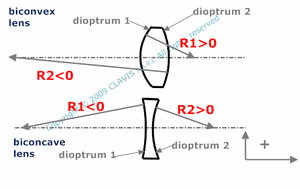As an example, for a biconvex lens, the radius of curvature R1 of the first surface ( or facet ) is positive and the radius of curvature R2 of its second surface is negative.

As another example, for a biconcave lens, the radius of curvature R1 of the first facet is negative and the radius of curvature R2 of the second facet is positive.A concave mirror has a negative radius of curvature R.
A convex mirror has a positive radius of curvature R.

Principal planes and focal pointsPrincipal planes are two planes perpendicular to the optical axis Oa. The secondary principal plane Hi is conjugated with the primary principal plane H by the optical system with a transverse magnification of +1. This means that an incident light ray intersecting H at the height h gives an emergent ray intersecting Hi at the same height h.
The primary principal point N and the secondary principal point Ni are the intersection of the optical axis respectively with H and Hi.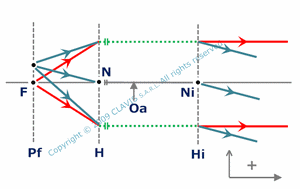Focal points are chracteristic points on the optical axis. The Image of the front focal point F by the optical system is located on Oa at infinity, which means that incident rays coming from F give emerging rays all parallel to Oa. The back focal point Fi is the image of the point located on Oa at infinity, which means that incident rays parallel to Oa give emerging rays all focusing on Fi.

F is the intersection of the optical axis with the front focal plane Pf. Pf is perpendicular to Oa and its image by the optical system is a plane at infinity. Accordingly, incident rays coming from a point of Pf and out of Oa give emerging rays all parallel but having a non null angle with Oa.Fi is the intersection of the optical axis with the back focal plane Pfi. Pfi is also perpendicular to Oa and is the image by the optical system of a plane located at infinity. Consequently, incident light rays all parallel and having a non null angle with Oa give emerging rays all focusing on a point of Pfi and out of Oa.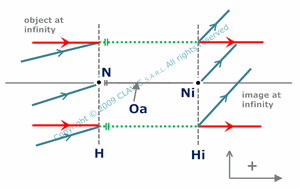Particular optical systems don't have focal points. They are called afocal systems. In this case, the image of an object at infinity is also at infinity. Afocal systems generate a constant angular magnification on an incident collimated beam. Their interest is to magnify object located far away from the observer ( binoculars, telescopes,...), expand laser beams, etc...

One of their particularity is to produce a constant transverse magnification whatever the object position.

Focal lengths and other parametersEffective focal length f in the object space is the algebric distance N F or H Pf.
Effective focal length fi in the image space is the algebric distance Ni Fi or Hi Pfi.

As principal planes are generally not coinciding with a facet, it is useful to calculate the front and back focal lengths ft and fb.
ft is the algebric distance from the first facet vertex to the front focal point F.

fb is the algebric distance from the last facet vertex to the back focal point Fi.

For refractive optical systems which object and image spaces have the same refraction index (for exemple a lens or a lenses assembly in the air or in another medium ), f = -fi. This is not the case for optical systems interfacing different media.

For reflective systems, f = fi.

*Considering the signs convention mentioned above and incident light rays from left to right, for converging refractive systems, fi is positive. For diverging refractive systems, fi is negative. For a converging mirror ( concave ) fi is negative. For a diverging mirror ( convex ) fi is positive.

Sometimes ( particulary in ophtalmic optic ), focal lengths are replaced by their inverse value : the power.

Some other characteristic lengths can be useful.

l : distance from the vertex of the first optical surface to the primary principal point N.
li : distance from the vertex of the last optical surface to the secondary principal point Ni.
d : distance from the primary principal plane H to the secondary principal plane Hi.

*See the tutorial "paraxial conjugation of optical systems"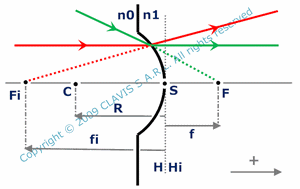A spherical refracting surface is a spherical surface interfacting two transparent different media. Primary and secondary principal planes are merging with the plane tangent to the surface and including its vertex. Focal lengths ( f, fi, ft and fb ) depend on the refraction index of the first medium, the refraction index of the second medium and the radius of curvature of the facet.

ft = f and fb = fi.For a thin lens, center thickness is neglected. Vertex of each surface and nodal points are assumed to coincide. Consequently, primary and secondary principal planes are merging. Focal lengths ( f, fi, ft and fb ) only depend on lens refraction index and radius of curvature of each facet.

ft = f = - fb = - fi.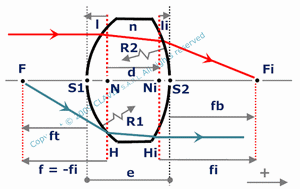Focal lengths ( f, fi, ft and fb ) depend on radius of curvature of each facet R1 and R2, refraction index n and center thickness e.

In the general case, front focal length ft and effective focal length in the object space f are different as well as back focal length fb and effective focal length in the image space fi.

f = - fi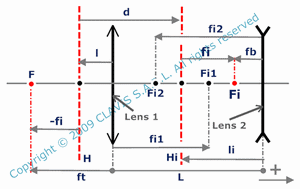Accordingly to thin lens approximation, ft is the distance from the first lens to the front focal point F of the system as well as fb is the distance from second lens to the back focal point Fi of the system.

Focal lengths of the system ( f, fi, ft and fb ) depend on lenses effective focal lengths fi1 and fi2 and distance between lenses L.

In the general case, the front focal length ft and the effective focal length in the object space f are different as well as the back focal length fb and the effective focal length in the image space fi. However, f = - fi.

When L is null, the system can be assumed to a thin lens. Then, ft = f = - fb = - fi.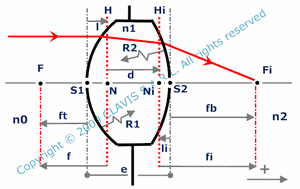Focal lengths (f, fi, ft and fb ) depend on lens and media refraction indexes ( n0, n1 and n2 ), radius of curvature of each facet ( R1 and R2 ) and center thickness e.

In the general case, f and ft are different as well as fi and fb.

Because n1 and n2 are different, f and -fi are different.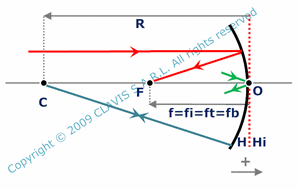Principal planes coincide with the plane containing the mirror vertex O and perpendicular to the optical axis. Focal lengths (f, fi, ft and fb ) are then identical and only depend on the radius of curvature of the mirror R.

The mirror center C is a characteristic point as all light rays coming from C are reflected by the mirror backward in the same direction.

The mirror vertex O is also characteristic as it reflects light rays in a symetrical direction regarding the optical axis.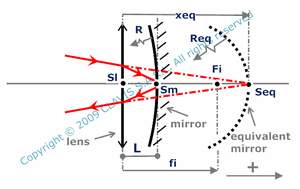Basic catadioptric systems are made of a refractive system and a mirror. Light goes first through the refractive system, is then reflected back by the mirror and finally goes through the refractive system a second time in the reverse way.

In its simplest configuration, a catadioptric system is made of a thin lens and a spherical mirror. The whole system is reflective and behaves like a virtual spherical mirror with an equivalent vertex Seq and an equivalent radius Req.

Req and the distance xeq from the lens to Seq depend on lens effective focal length fi, "real" mirror radius R and distance L between the lens and the "real" mirror vertex Sm.

References

"Optique Fondements et applications" - 2004 - author : José-Philippe Perez.

"Optique géométrique Imagerie et instruments" - 2007 - author : Bernard Balland.

"Optique géométrique paraxiale" - Institut d'optique théorique et appliquée - 1985 - author : Michel Cagnet.

"Formation des images Aberrations" - Institut d'optique théorique et appliquée - 1985 - author : Michel Cagnet.